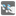• # mission idea "Wall Keeper"

## Question related to missionWall Keeper

This is a simple plan.

CheckiO has a lot of puzzle-solver missions. ( Sudoku Solver, Crossword Solver, 8 Puzzle Bulls and Cows Minesweeper )

I would like to add one.

Do you know Lights Out ? This is a simple and interesting puzzle.

How about implementing this solver in Python?

I would like to get a feedback about a mission I've just created.

sample of initial code:

```def wall_keeper(wall):
"""solver of Lights Out Puzzle

number of each panel
+--+--+--+--+--+
| 1| 2| 3| 4| 5|
+--+--+--+--+--+
| 6| 7| 8| 9|10|
+--+--+--+--+--+
|11|12|13|14|15|
+--+--+--+--+--+
|16|17|18|19|20|
+--+--+--+--+--+
|21|22|23|24|25|
+--+--+--+--+--+

:param:
e. g.
['00001',
'01000',
'00110',
'00100',
'00000']

1: Lighting up
0: Lights off

:return:
e. g.
[2, 6, 7, 8, 10, 12, 15, 18, 24, 25]
"""

return []

if __name__ == '__main__':
from itertools import chain

def checker(solution, wall):
w = [list(map(int, r)) for r in wall]
r, c = (a-1) // len(w), (a-1) % len(w)
w[r][c] = 1 - w[r][c]
if r+1 < len(w):
w[r+1][c] = 1 - w[r+1][c]
if r-1 > -1:
w[r-1][c] = 1 - w[r-1][c]
if c+1 < len(w):
w[r][c+1] = 1 - w[r][c+1]
if c-1 > -1:
w[r][c-1] = 1 - w[r][c-1]
return sum(chain(*w)) == 0

assert checker(wall_keeper, [
'00001',
'01000',
'00110',
'00100',
'00000']), 'wall_1'
```44